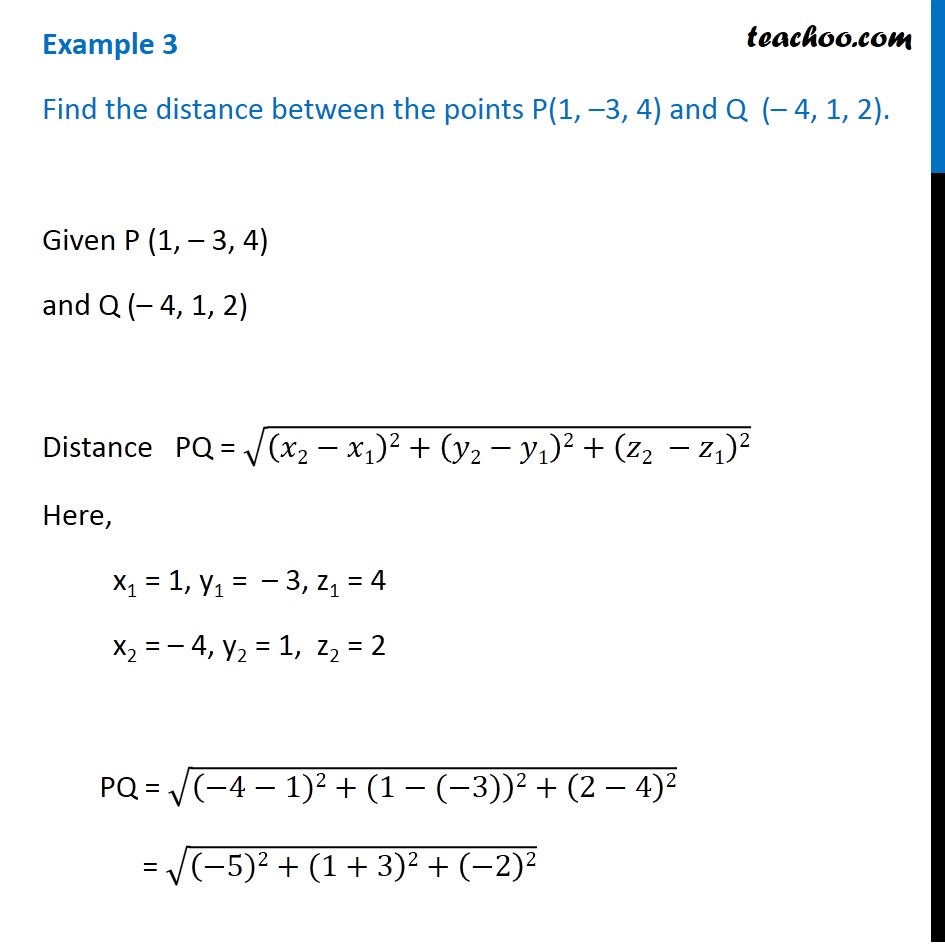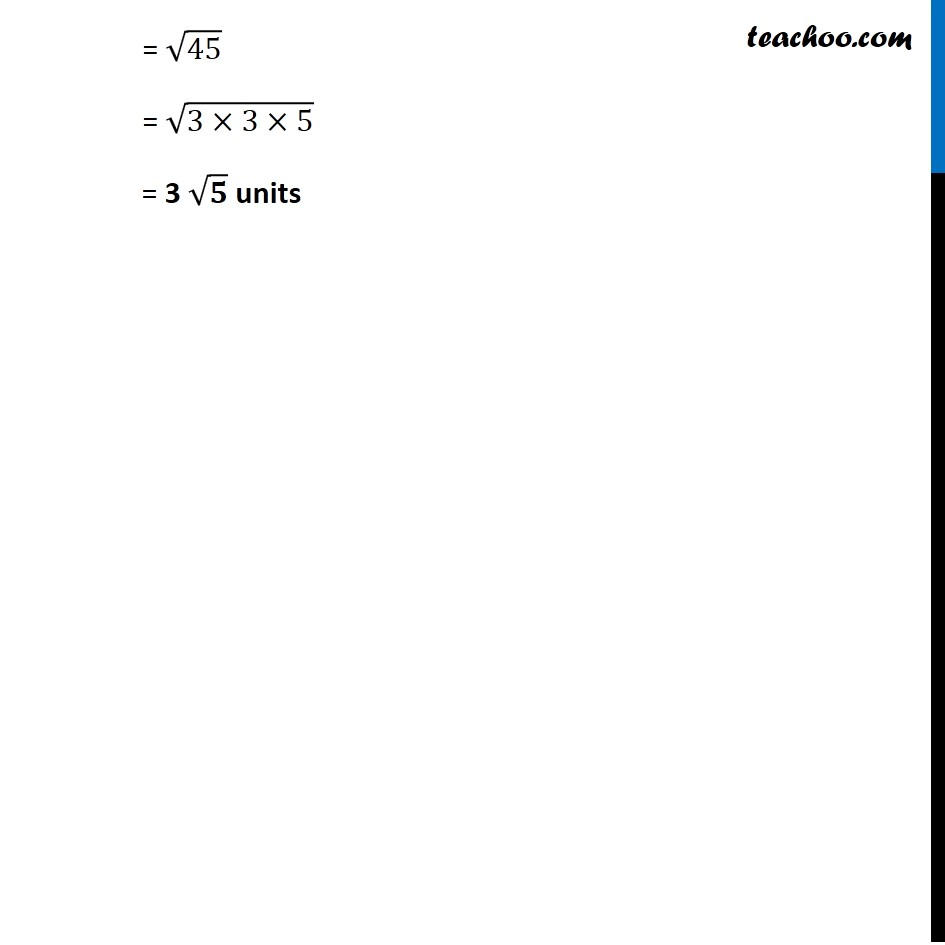Examples

Chapter 11 Class 11 - Intro to Three Dimensional Geometry
Serial order wiseLearn in your speed, with individual attention - Teachoo Maths 1-on-1 Class

### Transcript

Example 3 Find the distance between the points P(1, –3, 4) and Q (– 4, 1, 2). Given P (1, – 3, 4) and Q (– 4, 1, 2) Distance PQ = √((𝑥2−𝑥1)2+(𝑦2−𝑦1)2+(𝑧2 −𝑧1)2) Here, x1 = 1, y1 = – 3, z1 = 4 x2 = – 4, y2 = 1, z2 = 2 PQ = √((−4−1)2+(1−(−3))2+(2−4)2) = √((−5)2+(1+3)2+(−2)2) = √45 = √(3×3×5) = 3 √𝟓 units